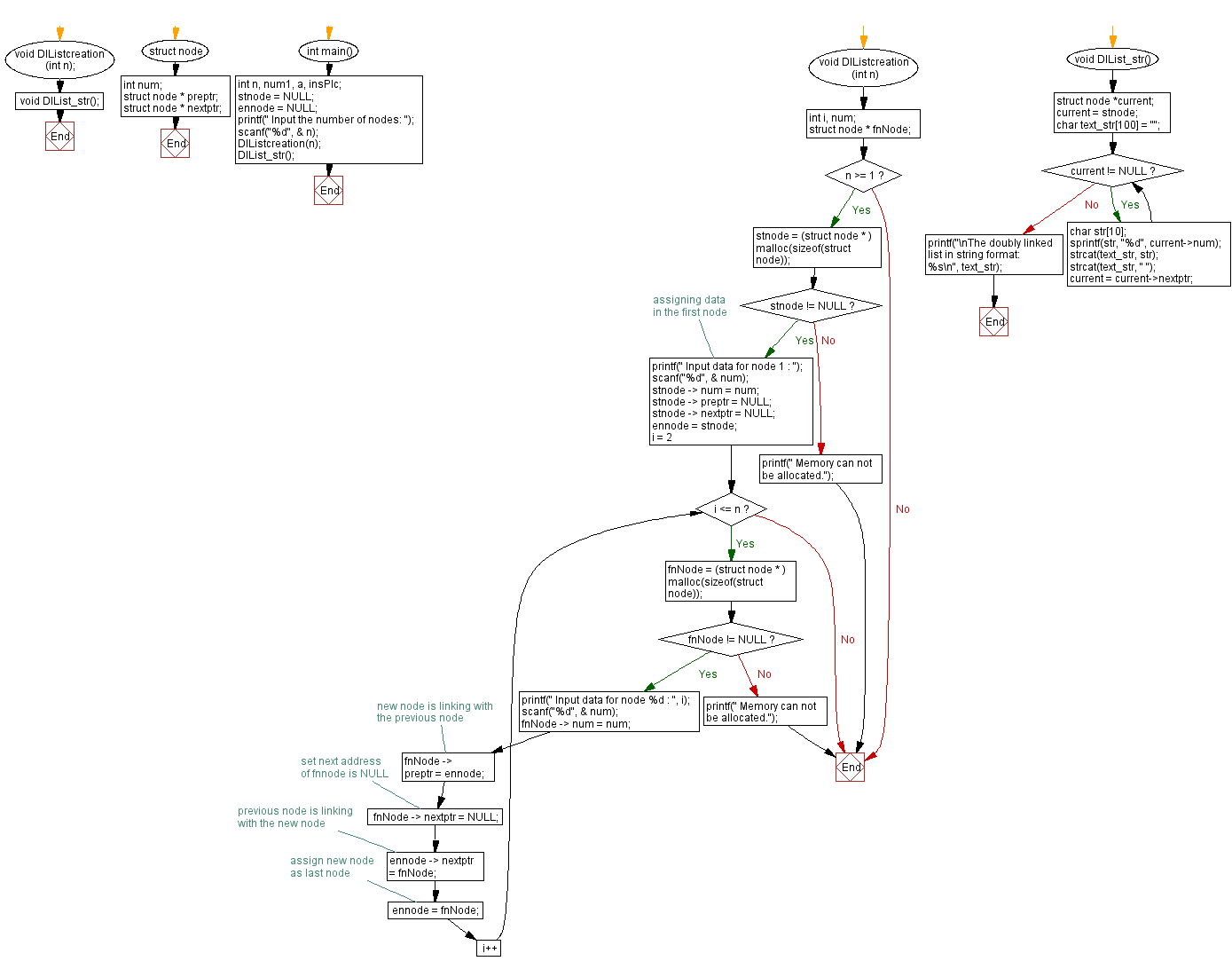﻿ C Program: Convert a Doubly Linked list into a string

# C Exercises: Convert a Doubly Linked list into a string

## C Doubly Linked List : Exercise-21 with Solution

Write a C program to convert a Doubly Linked list into a string.

Sample Solution:

C Code:

``````#include<stdio.h>
#include <stdlib.h>
#include <string.h>

struct node {
int num;
struct node * preptr;
struct node * nextptr;
}* stnode, * ennode;

void DlListcreation(int n);
void DlList_str();

int main() {
int n, num1, a, insPlc;
stnode = NULL;
ennode = NULL;
printf(" Input the number of nodes: ");
scanf("%d", & n);
DlListcreation(n);
DlList_str();
}
void DlListcreation(int n) {
int i, num;
struct node * fnNode;

if (n >= 1) {
stnode = (struct node * ) malloc(sizeof(struct node));
if (stnode != NULL) {
printf(" Input data for node 1 : "); // assigning data in the first node
scanf("%d", & num);
stnode -> num = num;
stnode -> preptr = NULL;
stnode -> nextptr = NULL;
ennode = stnode;
for (i = 2; i <= n; i++) {
fnNode = (struct node * ) malloc(sizeof(struct node));
if (fnNode != NULL) {
printf(" Input data for node %d : ", i);
scanf("%d", & num);
fnNode -> num = num;
fnNode -> preptr = ennode; // new node is linking with the previous node
fnNode -> nextptr = NULL; // set next address of fnnode is NULL
ennode -> nextptr = fnNode; // previous node is linking with the new node
ennode = fnNode; // assign new node as last node
} else {
printf(" Memory can not be allocated.");
break;
}
}
} else {
printf(" Memory can not be allocated.");
}
}
}

void DlList_str() {
struct node *current;
current = stnode;
char text_str = "";
while (current != NULL) {
char str;
sprintf(str, "%d", current->num);
strcat(text_str, str);
strcat(text_str, " ");
current = current->nextptr;
}
printf("\nThe doubly linked list in string format: %s\n", text_str);
}
```
```

Sample Output:

``` Input the number of nodes: 4
Input data for node 1 : 10
Input data for node 2 : 11
Input data for node 3 : 12
Input data for node 4 : 13

The doubly linked list in string format: 10 11 12 13

```

Flowchart :C Programming Code Editor:

What is the difficulty level of this exercise?

﻿